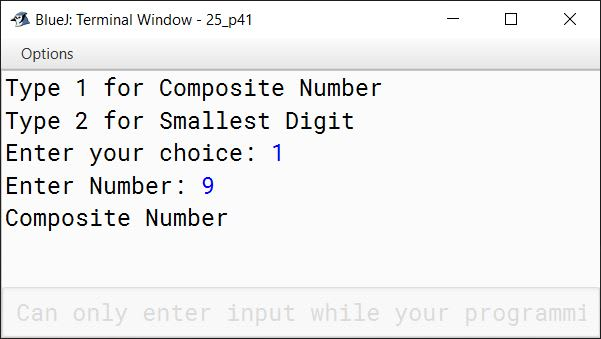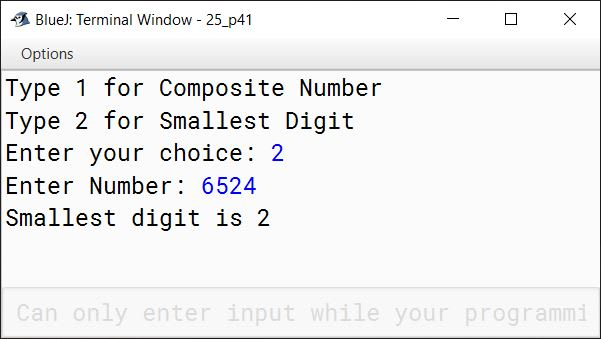# Solved 2013 Question Paper ICSE Class 10 Computer Applications

## Section A

#### Question 1

(a) What is meant by precedence of operators?

Precedence of operators refers to the order in which the operators are applied to the operands in an expression.

(b) What is a literal?

Literals are data items that are fixed data values. Java provides different types of literals like:

1. Integer Literals
2. Floating-Point Literals
3. Boolean Literals
4. Character Literals
5. String Literals
6. null Literal

(c) State the Java concept that is implemented through:

1. a superclass and a subclass.
2. the act of representing essential features without including background details.

1. Inheritance
2. Abstraction

(d) Give a difference between a constructor and a method.

Constructor has the same name as class name whereas function should have a different name than class name.

(e) What are the types of casting shown by the following examples?

1. double x = 15.2;
int y = (int) x;

2. int x = 12;
long y = x;

1. Explicit Type Casting
2. Implicit Type Casting

#### Question 2

(a) Name any two wrapper classes.

Two wrapper classes are:

1. Integer
2. Character

(b) What is the difference between a break statement and a continue statement when they occur in a loop?

When the break statement gets executed, it terminates its loop completely and control reaches to the statement immediately following the loop. The continue statement terminates only the current iteration of the loop by skipping rest of the statements in the body of the loop.

(c) Write statements to show how finding the length of a character array char[] differs from finding the length of a String object str.

Java code snippet to find length of character array:

char ch[] = {'x', 'y', 'z'};
int len = ch.length

Java code snippet to find length of String object str:

String str = "Test";
int len = str.length();

(d) Name the Java keyword that:

1. indicates that a method has no return type.
2. stores the address of the currently-calling object.

1. void
2. this

(e) What is an exception?

An exception is an abnormal condition that arises in a code sequence at run time. Exceptions indicate to a calling method that an abnormal condition has occurred.

#### Question 3

(a) Write a Java statement to create an object mp4 of class Digital.

Digital MP4 = new Digital();

(b) State the values stored in the variables str1 and str2:

String s1 = "good";
String s2 = "world matters";
String str1 = s2.substring(5).replace('t', 'n');
String str2 = s1.concat(str1);

Value stored in str1 is " manners" and str2 is "good manners". (Note that str1 begins with a space.)

##### Explanation

s2.substring(5) gives a substring from index 5 till the end of the string which is " matters". The replace method replaces all 't' in " matters" with 'n' giving " manners". s1.concat(str1) joins together "good" and " manners" so "good manners" is stored in str2.

(c) What does a class encapsulate?

A class encapsulates data and behavior.

(d) Rewrite the following program segment using the if..else statement:

comm = (sale > 15000)? sale * 5 / 100 : 0;

if(sale > 15000)
comm = sale * 5 / 100;
else
comm = 0;

(e) How many times will the following loop execute? What value will be returned?

int x = 2, y = 50;
do{
++x;
y -= x++;
}while(x <= 10);
return y;

The loop will run 5 times and the value returned is 15.

(f) What is the data type that the following library functions return?

1. isWhitespace(char ch)
2. Math.random()

1. boolean
2. double

(g) Write a Java expression for:

$ut + \dfrac{1}{2}ft^2$

u * t + 1 / 2.0 * f * t * t

(h) If int n[] = {1, 2, 3, 5, 7, 9, 13, 16}, what are the values of x and y?

x = Math.pow(n, n);
y = Math.sqrt(n + n);

x = Math.pow(n, n);
⇒ x = Math.pow(7, 3);
⇒ x = 343.0;

y = Math.sqrt(n + n);
⇒ y = Math.sqrt(9 + 16);
⇒ y = Math.sqrt(25);
⇒ y = 5.0;

(i) What is the final value of ctr when the iteration process given below, executes?

int ctr = 0;
for(int i = 1; i <= 5; i++)
for(int j = 1; j <= 5; j += 2)
++ctr;

The final value of ctr is 15.

The outer loop executes 5 times. For each iteration of outer loop, the inner loop executes 3 times. So the statement ++ctr; is executed a total of 5 x 3 = 15 times.

(j) Name the method of Scanner class that:

1. is used to input an integer data from the standard input stream.
2. is used to input a String data from the standard input stream.

1. nextInt()
2. nextLine()

## Section B

#### Question 4

Define a class named FruitJuice with the following description:

Data MembersPurpose
int product_codestores the product code number
String flavourstores the flavour of the juice (e.g., orange, apple, etc.)
String pack_typestores the type of packaging (e.g., tera-pack, PET bottle, etc.)
int pack_sizestores package size (e.g., 200 mL, 400 mL, etc.)
int product_pricestores the price of the product
Member MethodsPurpose
FruitJuice()constructor to initialize integer data members to 0 and string data members to ""
void input()to input and store the product code, flavour, pack type, pack size and product price
void discount()to reduce the product price by 10
void display()to display the product code, flavour, pack type, pack size and product price

import java.util.Scanner;

public class FruitJuice
{
private int product_code;
private String flavour;
private String pack_type;
private int pack_size;
private int product_price;

public FruitJuice() {
product_code = 0;
flavour = "";
pack_type = "";
pack_size = 0;
product_price = 0;
}

public void input() {
Scanner in = new Scanner(System.in);
System.out.print("Enter Flavour: ");
flavour = in.nextLine();
System.out.print("Enter Pack Type: ");
pack_type = in.nextLine();
System.out.print("Enter Product Code: ");
product_code = in.nextInt();
System.out.print("Enter Pack Size: ");
pack_size = in.nextInt();
System.out.print("Enter Product Price: ");
product_price = in.nextInt();
}

public void discount() {
product_price -= 10;
}

public void display() {
System.out.println("Product Code: " + product_code);
System.out.println("Flavour: " + flavour);
System.out.println("Pack Type: " + pack_type);
System.out.println("Pack Size: " + pack_size);
System.out.println("Product Price: " + product_price);
}

public static void main(String args[]) {
FruitJuice obj = new FruitJuice();
obj.input();
obj.discount();
obj.display();
}
}
##### Output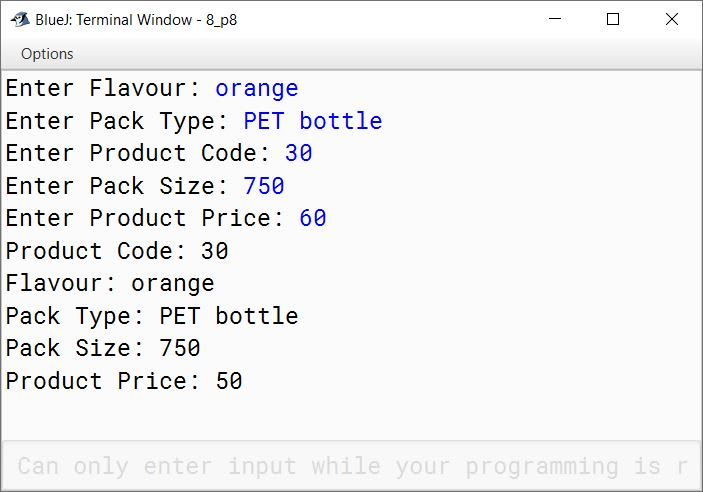#### Question 5

The International Standard Book Number (ISBN) is a unique numeric book identifier which is printed on every book. The ISBN is based upon a 10-digit code.

The ISBN is legal if:
1 × digit1 + 2 × digit2 + 3 × digit3 + 4 × digit4 + 5 × digit5 + 6 × digit6 + 7 × digit7 + 8 × digit8 + 9 × digit9 + 10 × digit10 is divisible by 11.

Example:
For an ISBN 1401601499
Sum = 1 × 1 + 2 × 4 + 3 × 0 + 4 × 1 + 5 × 6 + 6 × 0 + 7 × 1 + 8 × 4 + 9 × 9 + 10 × 9 = 253 which is divisible by 11.

Write a program to:

1. Input the ISBN code as a 10-digit integer.
2. If the ISBN is not a 10-digit integer, output the message "Illegal ISBN" and terminate the program.
3. If the number is divisible by 11, output the message "Legal ISBN". If the sum is not divisible by 11, output the message "Illegal ISBN".

import java.util.Scanner;

public class KboatISBNCheck
{
public static void main(String args[]) {
Scanner in = new Scanner(System.in);
System.out.print("Enter the ISBN: ");
long isbn = in.nextLong();

int sum = 0, count = 0, m = 10;
while (isbn != 0) {
int d = (int)(isbn % 10);
count++;
sum += d * m;
m--;
isbn /= 10;
}

if (count != 10) {
System.out.println("Illegal ISBN");
}
else if (sum % 11 == 0) {
System.out.println("Legal ISBN");
}
else {
System.out.println("Illegal ISBN");
}
}
}
##### Output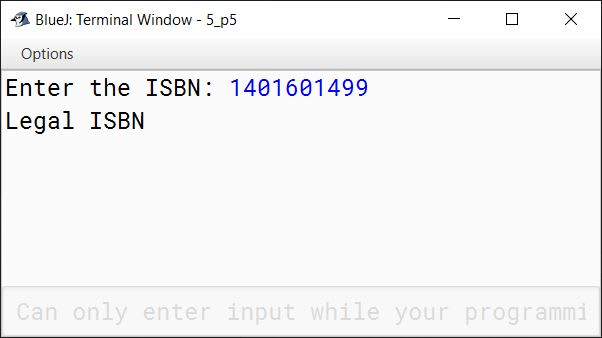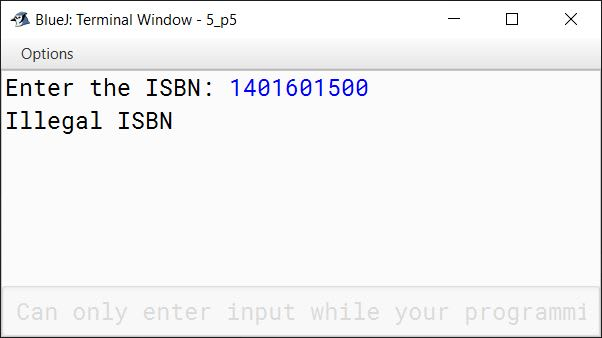#### Question 6

Write a program that encodes a word into Piglatin. To translate word into Piglatin word, convert the word into uppercase and then place the first vowel of the original word as the start of the new word along with the remaining alphabets. The alphabets present before the vowel being shifted towards the end followed by "AY".

Sample Input 1: London
Output: ONDONLAY

Sample Input 2: Olympics
Output: OLYMPICSAY

import java.util.Scanner;

public class KboatPigLatin
{
public static void main(String args[]) {

Scanner in = new Scanner(System.in);
System.out.print("Enter word: ");
String word = in.next();
int len = word.length();

word=word.toUpperCase();
String piglatin="";
int flag=0;

for(int i = 0; i < len; i++)
{
char x = word.charAt(i);
if(x=='A' || x=='E' || x=='I' || x=='O' || x=='U')
{
piglatin=word.substring(i) + word.substring(0,i) + "AY";
flag=1;
break;
}
}

if(flag == 0)
{
piglatin = word + "AY";
}
System.out.println(word + " in Piglatin format is " + piglatin);
}
}
##### Output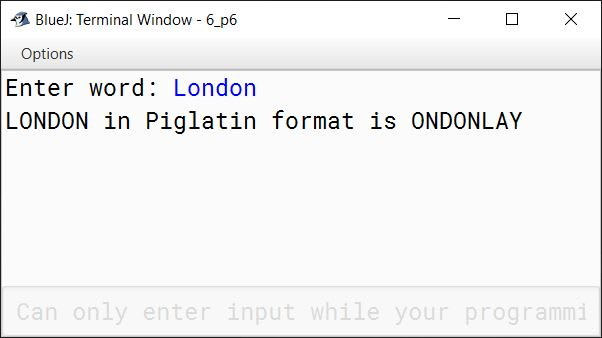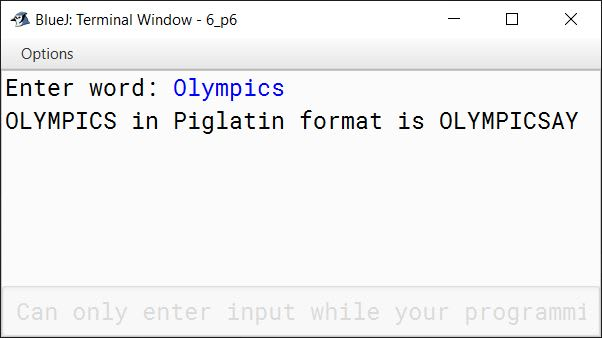#### Question 7

Write a program to input 10 integer elements in an array and sort them in descending order using bubble sort technique.

import java.util.Scanner;

public class KboatBubbleSortDsc
{
public static void main(String args[]) {
Scanner in = new Scanner(System.in);
int n = 10;
int arr[] = new int[n];

System.out.println("Enter the elements of the array:");
for (int i = 0; i < n; i++) {
arr[i] = in.nextInt();
}

//Bubble Sort
for (int i = 0; i < n - 1; i++) {
for (int j = 0; j < n - i - 1; j++) {
if (arr[j] < arr[j + 1]) {
int t = arr[j];
arr[j] = arr[j+1];
arr[j+1] = t;
}
}
}

System.out.println("Sorted Array:");
for (int i = 0; i < n; i++) {
System.out.print(arr[i] + " ");
}
}
}
##### Output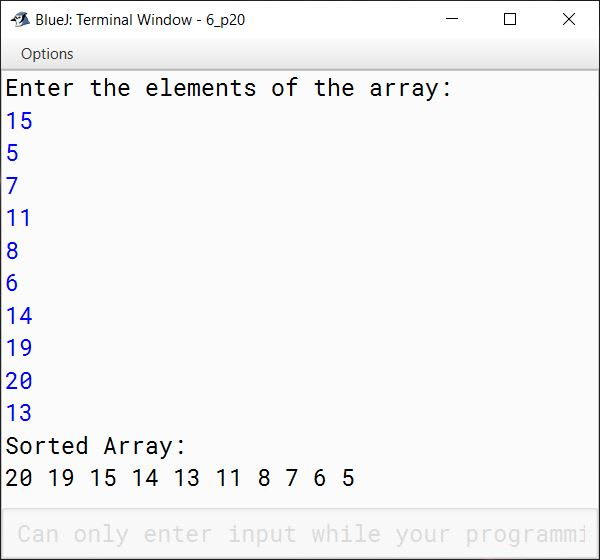#### Question 8

Design a class to overload a function series( ) as follows:

1. double series(double n) with one double argument and returns the sum of the series.
sum = (1/1) + (1/2) + (1/3) + .......... + (1/n)
2. double series(double a, double n) with two double arguments and returns the sum of the series.
sum = (1/a2) + (4/a5) + (7/a8) + (10/a11) + .......... to n terms

public class KboatSeries
{
double series(double n) {
double sum = 0;
for (int i = 1; i <= n; i++) {
double term = 1.0 / i;
sum += term;
}
return sum;
}

double series(double a, double n) {
double sum = 0;
int x = 1;
for (int i = 1; i <= n; i++) {
int e = x + 1;
double term = x / Math.pow(a, e);
sum += term;
x += 3;
}
return sum;
}

public static void main(String args[]) {
KboatSeries obj = new KboatSeries();
System.out.println("First series sum = " + obj.series(5));
System.out.println("Second series sum = " + obj.series(3, 8));
}
}
##### Output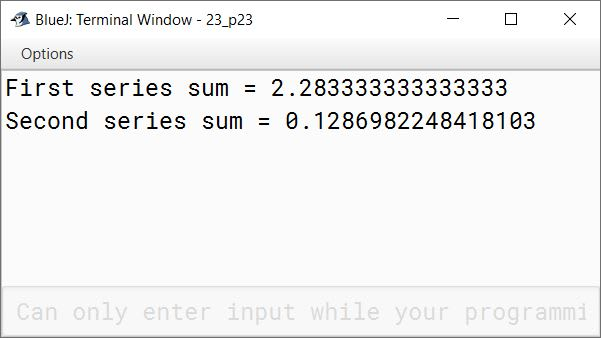#### Question 9

Using the switch statement, write a menu driven program:

1. To check and display whether a number input by the user is a composite number or not.
A number is said to be composite, if it has one or more than one factors excluding 1 and the number itself.
Example: 4, 6, 8, 9...
2. To find the smallest digit of an integer that is input:
Sample input: 6524
Sample output: Smallest digit is 2

For an incorrect choice, an appropriate error message should be displayed.

import java.util.Scanner;

public class KboatNumber
{
public static void main(String args[]) {
Scanner in = new Scanner(System.in);
System.out.println("Type 1 for Composite Number");
System.out.println("Type 2 for Smallest Digit");
int ch = in.nextInt();

switch (ch) {
case 1:
System.out.print("Enter Number: ");
int n = in.nextInt();
int c = 0;
for (int i = 1; i <= n; i++) {
if (n % i == 0)
c++;
}

if (c > 2)
System.out.println("Composite Number");
else
System.out.println("Not a Composite Number");
break;

case 2:
System.out.print("Enter Number: ");
int num = in.nextInt();
int s = 10;
while (num != 0) {
int d = num % 10;
if (d < s)
s = d;
num /= 10;
}
System.out.println("Smallest digit is " + s);
break;

default:
System.out.println("Wrong choice");
}
}
}
##### Output